The Covalent Bond Clarified Through the Use of the Virial Theorem

Frank Rioux
Department of Chemistry
St. John's University
College of St. Benedict
St.
Joseph, MN 56374

Abstract

Slater's method of analyzing the covalent bond using the virial theorem is presented for the hydrogen molecule. The overall conclusion reached is the same as that reached with Ruedenberg's well-known ab initio quantum mechanical study - electron kinetic energy plays an important role in chemical bond formation.

Introduction

In the second edition of his classic monograph, Molecular Quantum Mechanics, Peter Atkins begins the chapter on molecular structure with the following sentences (1),

Now we come to the heart of chemistry. If we can understand what holds atoms together as molecules we may also start to understand why, under certain conditions, old arrangements change in favor of new ones. We shall understand structure, and through structure, the mechanism of change.

Few will argue with Atkins' eloquent assertion that the chemical bond is at the heart of chemistry, however finding a satisfactory discussion of chemistry's central concept is difficult. For example, a survey of widely adopted general chemistry texts showed the following errors in the description of the covalent bond to be prevalent. Surprisingly the origin of some of these errors can be traced to two authoritative monographs on chemical bonding of enormous influence (2).

• The covalent bond is presented as a purely electrostatic phenomenon. Electron kinetic energy is never mentioned, even though the total energy of a molecule is a sum of kinetic and potential energy contributions, and atomic and molecular stability cannot be understood solely in terms of potential energy.
• Closely related to this is that what is actually an energy curve is called a potential energy curve. What is shown in introductory texts is the total molecular energy as a function of internuclear separation under the Born-Oppenheimer approximation. In other words, nuclear kinetic energy is frozen, but electron kinetic energy contributes to the total molecular energy.
• It is claimed that the electron density in the inter-nuclear (bond) region has a lower potential energy because it is attracted to two nuclei. Actually using simple electrostatic arguments it is easy to show that the electron-nuclear potential energy is higher in the internuclear region than it is closer to the nuclei. On the basis of potential energy alone the electrons would prefer to be in the nucleus.
• An energy minimum, or molecular ground state, is achieved because of increases in nuclear-nuclear and electron-electron repulsions as the internuclear separation decreases. As will be shown later, the immediate cause of the molecular ground state is a sharp increase in electron kinetic energy.
• The amount of electron density transferred to the bonding region is greatly overstated, sometimes implying that a pair of electrons is shared between two nuclei rather than by two nuclei.

Unfortunately, to find accurate analyses of the physical origin of the covalent bond one must go to the research or pedagogical literature; chemistry textbooks will not, in general, provide enlightenment. In the 1960s and 70s Ruedenberg and his collaborators carried out a detailed quantum mechanical study of the covalent bond in H2+ (4 - 6). The most important conclusion of this thorough and insightful study was that electron kinetic energy plays a crucial role in the formation of a chemical bond. Ruedenberg's contributions to the understanding of the chemical bond have been summarized in the pedagogical literature (7 - 12) and in review articles (13 - 15). There are also at least two encyclopedia entries which give accurate and clear interpretations of covalent bond formation (16, 17).

It is surprising that none of these efforts to make Ruedenberg's work accessible to the non-specialist have had any noticeable impact on the way chemical bonding is presented by the authors of chemistry textbooks currently used in the undergraduate curriculum. While physical chemistry texts avoid the errors cited above, they generally do not attempt to provide an "explanation" of the chemical bond. For example, after outlining the mathematical techniques required to solve Schrödinger's equation for H2+ and H2, physical chemistry texts do not interpret the calculations other than to say something to the effect that the stability of the chemical bond is a quantum mechanical effect that has no classical analog or explanation. Atkins' physical chemistry text is a notable exception; in a footnote he alerts the reader to the subtleties of the chemical bond and briefly outlines the interplay between kinetic and potential energy during the formation of the covalent bond in H2+(3).

It is not widely appreciated that John C. Slater used the virial theorem to come to a similar conclusion about the importance of electron kinetic energy in chemical bond formation in the early days of quantum mechanics. In a paper published in the inaugural volume of the Journal of Chemical Physics Slater pioneered the use of the virial theorem in interpreting the chemical bond. With regard to the virial theorem he said (18),

"... this theorem gives a means of finding kinetic and potential energy separately for all configurations of the nuclei, as soon as the total energy is known, from experiment or theory." (emphasis added)

The purpose of this paper is to outline Slater's method of analysis for the hydrogen molecule, the simplest example of the traditional two-electron chemical bond.

Background Theory

The Hamiltonian energy operator (in atomic units) for the hydrogen molecule consists of the following ten contributions,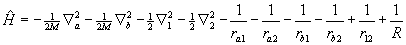Equation 1

where a and b label the nuclei, 1 and 2 label the electrons, and R = Rab, the internuclear separation. Under the Born-Oppenheimer approximation the nuclear kinetic energy operators drop out, and the molecular electronic energy as a function of internuclear separation, E(R), can be obtained by solving Schrödinger's equation variationally for the resulting eight-term electronic Hamiltonian, Hel, which in this analysis includes nuclear-nuclear repulsion.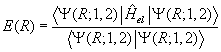Equation 2

Now one can return to equation (1) with the calculated E(R) and treat it as the molecular "potential" field in which the nuclei move. The nuclear kinetic energy operators are re-written in terms of the nuclear center-of-mass coordinate and the internal coordinate R. After discarding the term involving the motion of the center of mass, the Hamiltonian energy operator becomes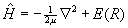Equation 3

Following Slater, a more empirical approach is taken here and the ab initio E(R) is replaced by an analytical surrogate such as the Morse function (19) which has a similar R-dependence (20).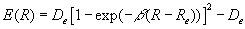Equation 4

In other words the Morse function represents the total energy of a diatomic molecule assuming the Born-Oppenheimer approximation.

Substitution of equation (4) into (3) yields an energy Hamiltonian for which Schrödinger's equation has an exact solution with the following quantized nuclear vibrational states (2),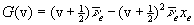Equation 5

where v = 0, 1, 2, ..., and the Morse parameters, De and b, are defined as,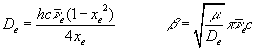Equation 6

Fitting equation (5) to spectroscopic data for H2 yields the following values for the Morse parameters: De = 7.92x10-19 joule; b = 0.0191 pm-1; and Re = 74.1 pm (21).

The Virial Theorem

At this point the virial theorem is used to obtain a total energy profile for covalent bond formation in H2. The first step in this approach is to acknowledge that the energy of a molecule is a sum of kinetic and potential energy contributions.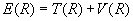Equation 7

The virial theorem for a diatomic molecule as a function of internuclear separation, R, is (14)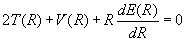Equation 8

Equations (7) and (8) can now be used to obtain expressions for the kinetic and potential energy in terms of the total energy and its first derivative.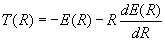Equation 9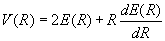Equation 10

Quoting Slater again (18)

These important equations determine the mean kinetic and potential energies as a function of r, one might almost say, experimentally, directly from the curves of E as a function of r which can be found from band spectra. The theory is so simple and direct that one can accept the results without question, remembering only the limitation of accuracy mentioned above. (Here Slater is refering to the neglect of zero point vibration in his analysis.)

Thus, when equation (4), parameterized as indicated above, is used in equations (9) and (10) the energy profile for covalent bond formation in H2 shown in Figure 1 is obtained.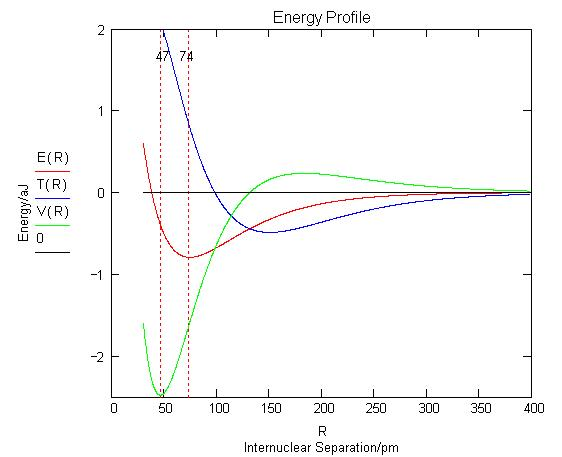Analysis

This energy profile shows that as the inter-nuclear separation decreases, the potential energy rises, falls, and then rises again. The kinetic energy first decreases and then increases at about the same value of R that the potential energy begins to decrease.

The quantum mechanical interpretation of the energy profile is as follows (4, 18). As the molecular orbital is formed at large R constructive interference between the two overlapping atomic orbitals on the hydrogen atoms draws electron density away from the nuclear centers into the inter-nuclear region. The potential energy rises as electron density is removed from the region around the nuclei, but the total energy decreases because of a larger decrease in kinetic energy due to charge delocalization - the electrons now belong to the molecule and not the individual atoms. Thus, a decrease in kinetic energy funds the initial build-up of charge between the nuclei that is traditionally associated with chemical bond formation.

Following this initial phase, at an inter-nuclear separation of about 150 pm, the potential energy begins to decrease and the kinetic energy increases, both sharply eventually, while the total energy continues to decrease gradually. This is an atomic affect, not a molecular one, as Ruedenberg clearly showed. The initial transfer of charge away from the nuclei and into the bond region allows the atomic orbitals to contract causing a large decrease in potential energy because the electron density is moved, on average, closer to the nuclei. The kinetic energy increases because the atomic orbitals are smaller and kinetic energy is inversely proportional to the square of the average orbital radius. This is an atomic affect because the orbital contraction actually causes some electron density to be withdrawn from the bonding region and returned to the nuclei.

An energy minimum is reached while the potential energy is still in a significant decline, indicating that kinetic energy, which is increasing rapidly, is the immediate cause of a stable bond and the molecular ground state in H2. The final increase in potential energy which is mainly due to nuclear-nuclear repulsion doesn't begin until the inter-nuclear separation is less than 50 pm, while the equilibrium bond length is 74 pm. Thus the common explanation that an energy minimum is reached because of nuclear-nuclear and electron-electron repulsion does not have merit. As Ruedenberg (4) has noted "there are no ground states in classical mechanics or electrostatics."

Conclusion

In conclusion a review of some basic principles and some additional observations are offered. Under the Born-Oppenheimer approximation the quantum mechanical energy operator for H2 consists of the final eight terms in equation (1), which fall into four types of energy contributions: electron kinetic energy, electron-electron, nuclear-nuclear, and electron-nuclear potential energy. Electron-nuclear potential energy is the only negative contribution to the total energy and is essential in understanding the stability of the chemical bond. H2 is a stable molecule because it has a lower total energy than its constituent atoms, not simply because there is a build-up of charge in the inter-nuclear region. As shown above, by itself this charge build-up actually increases the energy rather than decreasing it as is popularly, but incorrectly, believed.

We all recognize that electron kinetic energy is important at the computational level through its presence in the energy operator given in equation (1). In other words ground states are calculated by minimizing the total energy, the sum of the kinetic and potential energy contributions. However, many ignore kientic energy when it comes to interpretation because they believe it is irrelevant. The origin of this error is a common misapplication of the virial theorem.

For example, it is common to argue from the virial theorem in the form,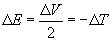Equation 11

that bond stability is due solely to potential energy, because it decreases with bond formation and kinetic energy actually increases by twice as much. However, this form of the virial theorem is valid only for R = Rºº and R = Re, the initial and final states. It tells us nothing about what is occuring when the bond is actually being formed - for that you need equations (7 - 10). As has been shown above, what is actually occuring during bond formation is a rather subtle role reversal between kinetic and potential energy. It is ironic that Slater correctly used the virial theorem to show that electron kinetic energy is essential to understanding the chemical bond in the 1930s, while today many use it incorrectly to show that electron kinetic energy is irrelevant.

It has been known since the early years of the 20th century that no dynamic or static array of charged particles is stable on the basis of classical electrostatic principles alone. Therefore, the quantum mechanical picture of a wave-particle duality for the electron and the peculiar quantum mechanical nature of kinetic energy are essential in understanding atomic and molecular stability and structure. The importance of kinetic energy runs counter to conventional opinion regarding the covalent bond in two seemingly paradoxical ways. First, a decrease in kinetic energy due to incipient molecular orbital formation funds the transfer of charge density into the internuclear region, lowering the total energy. Second, a large increase in kinetic energy associated with the subsequent atomic orbital contraction prevents the collapse of the molecule and causes an energy minimum and a stable molecular ground state.

In a previous publication (10b) the formation of the covalent bond in H2 was analyzed in terms of a two-step mechanism involving: a) orbital contraction ("hybridization") followed by b) orbital overlap (charge delocalization and redistribution). It was shown that step a) is an atomic and endothermic process, while b) is a molecular and exothermic effect. The present study is consistent with this prior analysis, but reverses the order of the contributing effects; atomic orbital overlap precedes atomic orbital contraction. Both mechanisms clearly reveal the important role that electron kinetic energy plays in chemical bond formation.

In summary I concur with Kutzelnigg's observation (22), "The chemical bond is a highly complex phenomenon which eludes all attempts at simple description." This means we must be careful how we teach the chemical bond to our students. It is not acceptable to present incorrect models of bonding to undergraduates because they are easier to understand and therefore easier to teach.

Literature Cited:

1. Atkins, P. W. Molecular Quantum Mechanics, Oxford University Press, Oxford, UK, 1983, p. 250.
2. Pauling, L. The Nature of the Chemical Bond, 3rd ed., Cornell University Press, Ithaca, 1960, pp. 19-21. Coulson, C. A. Valence, 2nd ed., Oxford University Press, London, 1961, pp. 85-86.
3. Atkins, P. W. Physical Chemistry, 6th ed., W. H. Freeman and Co., New York, 1998, p. 397.
4. Ruedenberg, K. Rev. Mod. Phys. 1962, 34, 326-352.
5. Feinberg, M. J.; Ruedenberg, K. J. Chem. Phys. 1971, 54, 1495-1511. Feinberg, M. J.; Ruedenberg, K. J. Chem. Phys. 1971, 55, 5804-5818.
6. Ruedenberg, K. In Localization and Delocalization in Quantum Chemistry; Chalvet, O. et al., Eds.; Reidel: Dordrecht, The Netherlands, 1975; Vol. I, pp 223-245.
7. Baird, N. C. J. Chem. Educ. 1986, 63, 660-664.
8. Harcourt, R. D. Am. J. Phys. 1988, 56, 660-661.
9. Nordholm, S. J. Chem. Educ. 1988, 65, 581-584.
10. Bacskay, G. G.; Reimers, J. R.; Nordholm, S. J. Chem. Educ. 1997, 74, 1494-1502.
11. Rioux, F. a) Chem. Educator 1997, 2(6), 1-14; b) Chem. Educator 2001, 6(5), 288-290.
12. Harcourt, R. D.; Solomon, H.; Beckworth, J.; Chislett, L. Am. J. Phys. 1982, 50, 557-559.
13. Kutzelnigg, W. Angew. Chem. Int. Ed. Eng. 1973, 12, 546-562.
14. Melrose, M. P.; Chauhan, M.; Kahn, F. Theor. Chim. Acta 1994, 88, 311-324.
15. Gordon, M. S.; Jensen, J. H. Theor. Chem. Acc. 2000, 103, 248-251.
16. Gordon, M. S.; Jensen, J. H. Encyclopedia of Computational Chemistry, Schleyer, P. v. R., Ed.; John Wiley & Sons: New York, 1998, pp 3198-3214.
17. Harris, F. E. In Encyclopedia of Physics, 2nd Edition; Lerner, R. G.; Trig, G. L., Eds.; VCH Publishers, Inc.: New York, 1991, pp 762-764.
18. Slater, J. C. J. Chem. Phys. 1933, 1, 687-691.
19. Morse, P. M. Phys. Rev. 1929, 34, 57.
20. For a virial theorem analysis using the best ab initio results for H2 see, Winn, J. S. J. Chem. Phys. 1981, 74, 608-611.
21. Huber, K. P.; Herzberg Molecular Spectra and Molecular Structure, vol IV, Constants of Diatomic Molecules; Van Nostrand Reinhold: New York, 1979.
22. Kutzelnigg, W. Angew. Chem. Int. Ed. Eng. 1984, 23, 292.

Back to Frank Rioux's homepage.